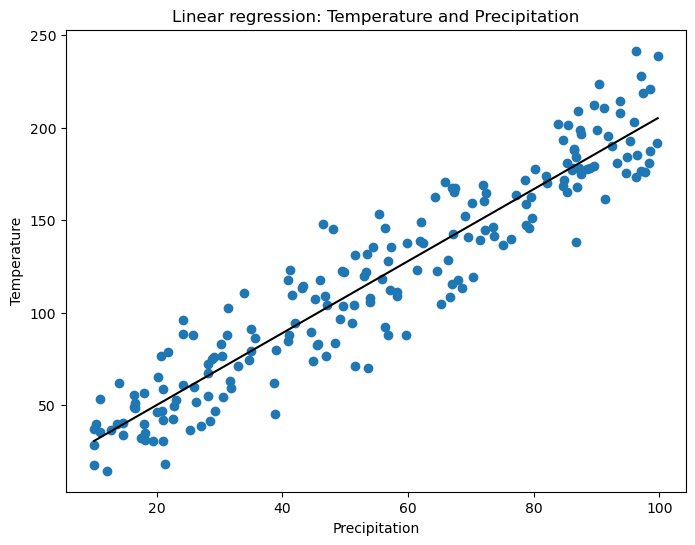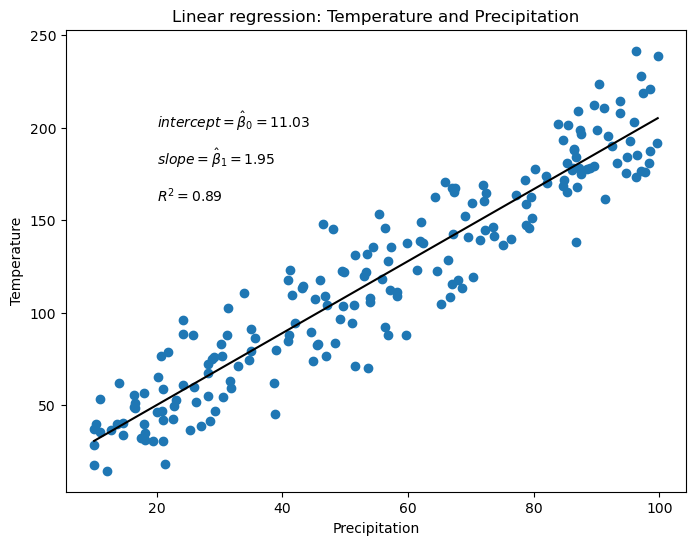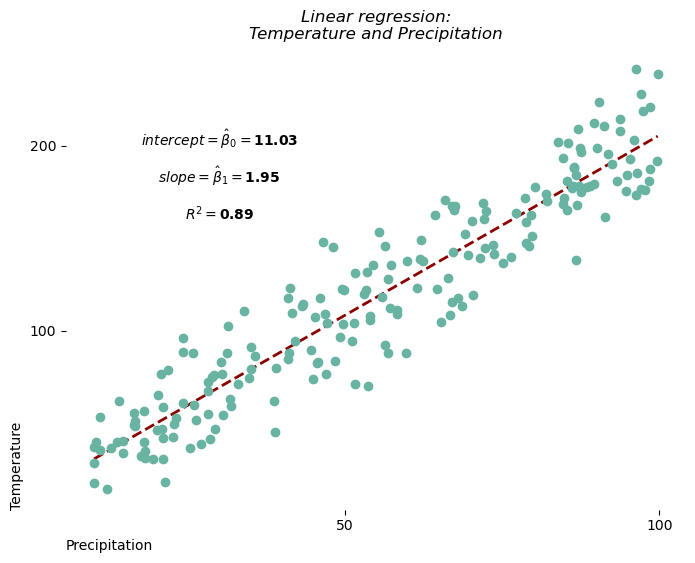The linear regression is used to model the relationship between a numerical variable and several other variables. In the case of a relationship between 2 variables, we may decide to display our model on top of a scatter plot to illustrate how well the model fits the data.
With matplotlib, you can easily create a scatter plot with a linear regression on top. We'll also see how to display statistical results such as R-squared directly on the graph. Our model will be created using the scikit-learn python library.

## Libraries

First, you need to install the following librairies:

• matplotlib is used for plot creating the charts
• pandas is used to put the data into a dataframe
• scikit-learn is used to create the linear regression
• numpy is used to generate some data

The linear regression will be create using scikit-learn: install it using the pip install -U scikit-learn command

import pandas as pd
import matplotlib.pyplot as plt
import numpy as np
from sklearn.linear_model import LinearRegression

## Dataset

In this post, we'll use fake weather data about temperature and precipitation.

• We use the random.normal() and random.uniform() functions from numpy to generate data
• Then we use pandas to put these variables into a dataframe
sample_size = 200
precipitation = np.random.uniform(10, 100, sample_size)
temperature = 2*precipitation + np.random.normal(10, 20, sample_size)

df = pd.DataFrame({'temp': temperature,
'prec': precipitation})

## Get model parameters

First, we'll start by retrive the values we want. For this, we need to use the LinearRegression() object from scikit-learn and fit it to our data.

This post does not cover any statistical/math details

# Define predictive and target variables
x = df['prec']
x = np.array(x).reshape((-1, 1)) # we need to work with a np array and to reshape it

y = df['temp']

# Create and fit model
model = LinearRegression()
model.fit(x, y)
LinearRegression()

Now that we have our model, let's get our coefficients and R squared!

r2 = model.score(x,y)
coefficients = model.coef_
intercept = model.intercept_

## Scatter plot with linear regression line

Now let's use the stats we got above and add them to the plot of scatter plot of each group using the text() function from matplotlib

# Init plots
fig, ax = plt.subplots(figsize=(8,6))

ax.scatter(df['prec'], df['temp'])

# Define parameters of the regression line
num = len(df)
start = df['prec'].min()
end = df['prec'].max()
xseq = np.linspace(start, end, num=num)

# Plot the line
ax.plot(xseq, intercept+coefficients*xseq, color="black", lw=1.5)

# Add a title and axis label
ax.set_title('Linear regression: Temperature and Precipitation')
ax.set_xlabel('Precipitation')
ax.set_ylabel('Temperature')

# Show the plot
plt.show()## Scatter plot with estimated parameters

Now let's use the stats we got above and add them to the plot using the text() function from matplotlib.

For example, to display the value of the intercept calculated by the model (usually called β^0\hat\beta_0), we create a string beta0, which is the sum of a string written in Latex (part of the string between $) and the value of the calculated intercept rounded to 2 decimal places. And then we do the same thing for the slope and the R2R^2. # Init plots fig, ax = plt.subplots(figsize=(8,6)) ax.scatter(df['prec'], df['temp']) # Define parameters of the regression line num = len(df) start = df['prec'].min() end = df['prec'].max() xseq = np.linspace(start, end, num=num) # Plot the line ax.plot(xseq, intercept+coefficients*xseq, color="black", lw=1.5) # Add the parameters beta0 = r'$intercept = \hat\beta_0 =$' + str(round(intercept,2)) ax.text(20, 200, beta0, fontsize=10) beta1 = r'$slope = \hat\beta_1 =$' + str(round(coefficients,2)) ax.text(20, 180, beta1, fontsize=10) r_squared = r'$R^2 =' + str(round(r2,2)) ax.text(20, 160, r_squared, fontsize=10) # Add a title and axis label ax.set_title('Linear regression: Temperature and Precipitation') ax.set_xlabel('Precipitation') ax.set_ylabel('Temperature') # Show the plot plt.show()## Custom the chart and the slope Using what we've just seen and other features, we're going to customize our graphic to make it even more aesthetic! • add zorder argument to the scatter() and plot() function to specify that markers should be on top of the line • change color of the markers via the color argument • put the text in bold using the weight='bold' argument • dash the line with the linestyle="--" argument • remove axis and spines • center the texts with the horizontalalignment='center' argument # Init plots fig, ax = plt.subplots(figsize=(8,6)) ax.scatter(df['prec'], df['temp'], color='#69b3a2', zorder=2) # Define parameters of the regression line num = len(df) start = df['prec'].min() end = df['prec'].max() xseq = np.linspace(start, end, num=num) # Plot the line ax.plot(xseq, intercept+coefficients*xseq, color="darkred", lw=2, zorder=1, linestyle="--") # Add the parameters beta0 = r'intercept = \hat\beta_0 =' + str(round(intercept,2)) ax.text(30, 200, beta0, fontsize=10, weight='bold', horizontalalignment='center') beta1 = r'slope = \hat\beta_1 =' + str(round(coefficients,2)) ax.text(30, 180, beta1, fontsize=10, weight='bold', horizontalalignment='center') r_squared = r'R^2 =\$' + str(round(r2,2))
ax.text(30, 160, r_squared, fontsize=10, weight='bold', horizontalalignment='center')

# Remove axis labels
ax.set_xticks([50, 100])
ax.set_yticks([100, 200])

# Removes spines
ax.spines[['right', 'top', 'left', 'bottom']].set_visible(False)

# Add a title and axis label
ax.set_title('Linear regression:\nTemperature and Precipitation',
style='italic')
ax.set_xlabel('Precipitation', loc='left')
ax.set_ylabel('Temperature', loc='bottom')

# Show the plot
plt.show()## Going further

This post explains how to represent the results of a linear regression in a scatter plot.

For more examples of charts with statistics, see the statistics section. You may also be interested in how to create a highly cutomized scatter plot with regression

## Contact & Edit

👋 This document is a work by Yan Holtz. You can contribute on github, send me a feedback on twitter or subscribe to the newsletter to know when new examples are published! 🔥